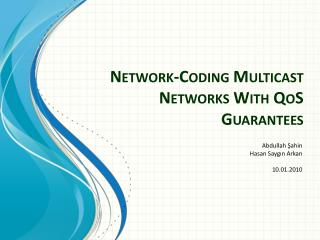DownloadDownload PresentationNetwork-Coding Multicast Networks With QoS Guarantees

# Network-Coding Multicast Networks With QoS Guarantees

Télécharger la présentation## Network-Coding Multicast Networks With QoS Guarantees

- - - - - - - - - - - - - - - - - - - - - - - - - - - E N D - - - - - - - - - - - - - - - - - - - - - - - - - - -
##### Presentation Transcript

1. Network-Coding Multicast Networks With QoS Guarantees Abdullah Şahin Hasan Saygın Arkan 10.01.2010

2. Outline

3. What we are going to present …

4. Define The Problem …

5. Solve for Unicast

6. Convert to Multicast

7. Introduction

8. Introduction • “Network-Coding Multicast Networks With QoSGuarantees” • Xuan, Y.: Lea, C.-T. • IEEE/ACM Transactions on Networking • 30 August 2010 • Related Work • Terms • QoS, Network Coding, unicast, multicast…

9. Unicast & Multicast Congession

10. Problem Definition • Admission Control – How? • New QoS Architecture – Non-Blocking Network! • No admission control • Low throughput for multicast • Impractical • Data Transmission • Transmission in Client – Local Server TRIVIAL • Transmission in Backbone PROBLEM!

11. Problem Definition • Transmission in Backbone PROBLEM!

12. Unicast Data Packet Data Packet

13. Multicast Data Packet Data Packet Data Packet Data Packet

14. Unicast Solution • tij= traffic rate from i edge to j edge • αi= ingress traffic & βi = egress traffic • (αi, βi) = (Θ αi’ , Θ βi’) • Task is maximizing Θ Edge Router αi= ingress traffic βi = egress traffic

15. Unicast Solution • Σtij< αi’ • Σtij< βi’ • Not Applicable on Multicast • α = β for unicast, but not for multicast Edge Router

16. Multicast Solution G = multicast edge group = { sg, D(g), tg } source, destination set, data rate Binary Vectors: ϒg(i) = 1, if i = sgδg(j) = 1, if j € D(g) 0, otherwise 0, otherwise

17. Multicast Solution • Σϒg(i) . tg< αi’ - ingress traffic • Σδg(j). tg< βi’ - egress traffic • tij = Σ(δg(j) .ϒg(i) . tg)

18. Optimal Routing j i xije

19. Optimal Routing

20. Optimal Routing

21. Optimal Routing • For IP networks • Calculation on weights • MPLS-Type Explicit Routing Networks • Arbitrarily chosen nodes, and calculation of max loaded link

22. Numerical Results

23. Numerical Results • Constraint-Based Routing Approach • Non-Blocking Based Approach • 15 Nodes, 62 directed links, capacity of 300. • 10 consecutive rejects = fully loaded • Number of receivers per multicast flow is random (binomial distribution [2, N-1] , N is total edge

24. Numerical Results • Nonblocking Multicast Networks • b/a ratio, average fan-out = 3, 15 edge nodes

25. Numerical Results • Nonblocking Multicast Networks • b/a ratio, average fan-out = 4, 15 edge nodes

26. Numerical Results • Nonblockingvs CBR • 5 edge nodes, average fan-out = 3

27. Numerical Results • Nonblockingvs CBR • 15 edge nodes, average fan-out = 3

28. Numerical Results • Nonblockingvs CBR • 15 edge nodes, average fan-out = 4

29. Conclusion • Better to have admission control at the edge, NOT inside it! • Non-Blocking removes that need • Main Problem – low throughput • Optimal Paths in Unicast = Optimal Paths in Multicast Nonblocking with Network Coding

30. Questions?